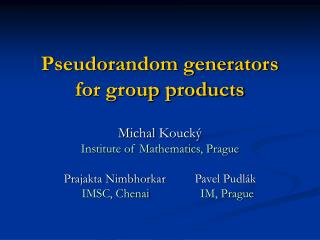Download PresentationPseudorandom generators for group productsPseudorandom generators for group products - PowerPoint PPT Presentation

Download PresentationPseudorandom generators for group products
An Image/Link below is provided (as is) to download presentation

Download Policy: Content on the Website is provided to you AS IS for your information and personal use and may not be sold / licensed / shared on other websites without getting consent from its author. While downloading, if for some reason you are not able to download a presentation, the publisher may have deleted the file from their server.

- - - - - - - - - - - - - - - - - - - - - - - - - - - E N D - - - - - - - - - - - - - - - - - - - - - - - - - - -
Presentation Transcript

1. Pseudorandom generators for group products Michal Koucký Institute of Mathematics, Prague Prajakta Nimbhorkar Pavel Pudlák IMSC, Chenai IM, Prague

2. 1 0 Branching programs 0 i 1 1 j 0 w t • pij = Pr[ reaching j from i ] • models randomized space bounded computation • space s → width w ≈ 2O( s )

3. Goal: Estimate probabilities pij (up-to additive error ε) in small space. Possible solution: Find a small set F  {0,1}t so that pij’s are well approximated by taking a random path according to a random sample from F. Want: A single set F working for all branching programs of length n, width n, and all i and j. → a random set F of size 2O(log n + log 1/ε) will do.

4. Goal: Find an explicit set F  {0,1}n , i.e., F : {0,1}l→ {0,1}n computable in small space, where l ≈ O(log n + log 1/ε). Our result: Explicit F : {0,1}l→ {0,1}n , where l = O( (2O(w log w) + log 1/ε) ∙ log n ) that works for all permutation branching programs of width w and length n. • permutation b.p. … in each layer the 0-edges form a permutation and 1-edges form a permutation.

5. Equivalent formulation for group products [MZ]: A fixed group G and elements g1, g2, …, gn G approximate the distribution R on G given by where r1, r2, … rnR {0,1} We have: F : {0,1}l→ {0,1}n so that r1, r2, …, rn given by the output of F approximate R well for any choice of g1, g2, …, gn G. l = O( (|G|O(1)+ log 1/ε) ∙ log n ) • For G=({0,1},+) →ε-biased spaces. r1 r2rn g1 ∙ g2 ∙ ∙ ∙ gn

6. Known results: • width n and length n • [Nisan92] l = O( log2n ) • [INW94] l = O( log2n ) • width w and length n (permutation/regular) • [BV10] l = O( (w4 log log n + log 1/ε ) log n ) • [BRRY10] l = O( (log w + log log n + log 1/ε ) log n) • ours l = O( (2O(w log w) + log 1/ε) log n ) • other combinatorial structures • [LRTV10, MZ09, GMRZ11] l = O( log n + logO(1) 1/ε) cyclic groups • …

7. Techniques: Convolution * R1, R2 probability distributions on G R1* R2 probability distribution on G s.t. for any g  G R1 *R2 (g) = ∑h G R1(h) ∙ R2(h-1 g) Examples: r1 rn/2 rn/2+1 rn g1 ∙ ∙ ∙ gn/2 * gn/2+1 ∙ ∙ ∙ gn r1 r2 rn g1 * g2 * * gn

8. a1 an/2 an/2+1 an g1 ∙ ∙ ∙ gn/2 * gn/2+1 ∙ ∙ ∙ gn Recursive convolution (~INW): D1 D2 a1… an/2 and an/2+1 … an obtained using Fn/2: {0,1}l→ {0,1}n/2 • Fn (s,s’) = Fn/2(s) ◦ Fn/2(s’) → D1 * D2 leads to Fn: {0,1}O( n )→ {0,1}n • Fn (s,d) = Fn/2(s) ◦ Fn/2( s(d) ) → D1 *γ D2 leads to Fn: {0,1}O( k log n)→ {0,1}n s(d) … d-th neighbor of s in a k-regular expander on 2l vertices

9. D1 *γ D2 D1 * D2 – D1 *γ D2 < γ Thm: If R1, R2, … RN are distributions obtained from group products, F is a formula built from R1, R2, … RN using *, and F’ is obtained from F by replacing * with *γ then  DF – DF’ < γ 2c|G|11 * *γ R1 * R1 *γ * R4 *γ R4 R2 R3 R2 R3 F F’

10. Proof ideas: D1, D2, R1, R2 distr. on G D1 = R1 + ε1 D2 = R2 + ε2 where ∑hGε1(h) = 0 ∑hGε2(h) = 0 • D1 * D2 = R1 * R2 + ε1 * R2 + R1 * ε2 +ε1 * ε2 • D1 *γ D2 = … +εγ where εγ < γ • If R2 is uniform then ε1 * R2 = 0. • If R2 is close to uniform then ε1 * R2 is close to 0. • If the support of R2 is the whole group G thenε1 * R2 < (1-δ) ε1 .

11. Open problems • Improve dependence on the width of the branching program/group size, and on the error ε. • Remove restrictions on the branching programs